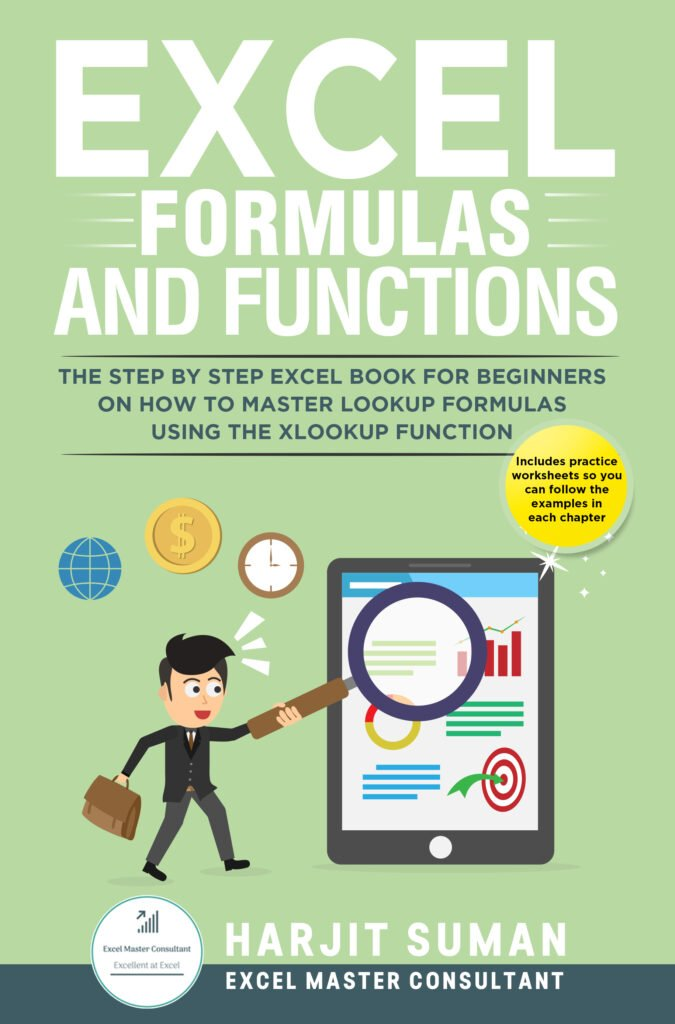# Excel Formulas and Functions: The Step by Step Excel Book for Beginners on how to Master Lookup Formulas using the XLOOKUP FunctionPart of the Excel Formulas and Functions series:
Editions:Paperback: £ 6.99
ISBN: B08F8193G6
Size: 15.24 x 22.86 cm
Pages: 82
Kindle: £ 2.99
ISBN: B08F73L8V5
Pages: 87

★★★ Create Powerful and Versatile Lookup Formulas by Mastering the new XLOOKUP Function ★★★

Over the years, Microsoft release new tools and Excel functions to make Excel the biggest and best spreadsheet application in the world. One new feature is the XLOOKUP function. If you have ever used VLOOKUP and HLOOKUP, you will know how great they are but you may also be aware of their limitations. With XLOOKUP, Microsoft have addressed these limitations to make it the most versatile and flexible Excel lookup function to date.

#### WHAT YOU WILL LEARN FROM READING THIS BOOK

Excel Formulas and Functions: The Step by Step Excel Book for Beginners on how to Master Lookup Formulas using the XLOOKUP Function is the most comprehensive book yet on how to write XLOOKUP formulas.

At the beginning of this Excel book you will learn what XLOOKUP is, what its syntax and arguments are and why it is better than the most popular lookup function, VLOOKUP. One of the key things you need to know about how to write excel formulas is understanding what relative and absolute cell references are. In this Excel book, you will learn what they are and when and how to use them so your excel formulas do not return errors or incorrect results. In the middle and end sections of this book, you will be given many examples of how to use XLOOKUP. You will learn how to create vertical and horizontal lookups, how to trap any errors that may occur, how to perform an approximate match, how to perform partial matches and how to extract the last value in a column or array. You will also learn how to return values in multiple cells using just one XLOOKUP formula and how to perform complex two-way lookups easily. You can also follow the examples in each chapter by downloading the free practice worksheets.

Here are some of the topics you will learn from this Excel book:

• What is XLOOKUP?
• What the XLOOKUP syntax is and an explanation of all its arguments
• What the limitations of VLOOKUP are and how XLOOKUP addresses these
• What are relative and absolute cell references
• How to perform vertical lookups using XLOOKUP
• How to perform horizontal lookups using XLOOKUP
• What the different error types are in Excel
• How to use XLOOKUP to replace errors with more meaningful values
• How to perform an approximate match using XLOOKUP
• How to use wildcard characters to perform partial matches with XLOOKUP
• How to extract the last value in a column or array using XLOOKUP
• Learn what the new ‘Spill’ term is and why it occurs
• How to return multiple values across cells using just one XLOOKUP formula to save time
• How to perform two-way lookups using XLOOKUP which replaces the more complex INDEX+MATCH functions

#### HOW YOU WILL BENEFIT AFTER READING THIS BOOK?

Once you have read this Excel book you will know how to perform complex lookups to extract information from your data set quickly and easily. This book will save you time and effort and will take your Excel skills to the next level. You will see the benefits of using XLOOKUP over other lookup functions such as VLOOKUP, HLOOKUP, LOOKUP and INDEX+MATCH functions and realise just how flexible and versatile this function is over other lookup functions which will ultimately make you more efficient.

Many workplaces require you to work with large data sets and to manipulate and extract information from this data set. There is no easier way to do this than with XLOOKUP. This book will teach you how.

Excerpt:
Reviews:Andrew Richmond on Amazon wrote:

★★★★★ Invaluable!

This is now my go to for Excel. Really useful and easy to follow. Helpful for beginners and advanced users, alike.